# 更高效能表現、更低資源佔用，高精度計算資料型別 DecimalV3 揭祕

• 金融行業：在金融交易中經常涉及到小數，比如利息、金額的計算，金融場景對數字準確的要求極高，因此精確的小數運算是必要的。
• 財務軟體：財務軟體通常需要進行復雜的財務計算，Decimal 型別可以提供精確的小數計算，避免計算過程中產生的舍入誤差。
• 科學計算、工程計算等其他場景。

### DecimalV3 功能介紹

Apache Doris 1.2.1 之前的版本中，我們已對 Decimal(precision, scale)(precision<=27) 資料型別進行了支援，隨著 Apache Doris 使用者的持續增長，銀行、證券、基金等金融領域的使用者也隨之快速增長，對高精度的小數計算場景也提出了更高的要求，舊的 Decimal 資料型別已無法滿足。因此，我們在 Apache Doris 1.2.1 推出了精度更高、速度更快的 DecimalV3(precision, scale)(precision<=38)，實現了真正意義上的高精度定點數，相比於老版本中的 Decimal ，DecimalV3 有以下核心優勢：

1. 可表示範圍更大。DECIMALV3 對 Precision 和 Scale 的取值範圍進行擴充。
2. 記憶體佔用更低，效能更高。老版本的 Decimal 需要佔用 16 Bytes 的記憶體，而 DecimalV3 對記憶體可進行自適應調整，如下所示。
``````+----------------------+-------------------+
|     precision        | 佔用空間（記憶體/磁碟）|
+----------------------+-------------------+
| 0 < precision <= 8   |      4 bytes      |
+----------------------+-------------------+
| 8 < precision <= 18  |      8 bytes      |
+----------------------+-------------------+
| 18 < precision <= 38 |     16 bytes      |
+----------------------+-------------------+
``````
1. 更完備的精度推演。

### 精度推演規則

DECIMALV3 有一套很複雜的型別推演規則，針對不同的表示式，會應用不同規則進行精度推演，下面來介紹一下推演規則：

1. 四則運算
• 加法 / 減法：DECIMALV3(a, b) + DECIMALV3(x, y) -> DECIMALV3(max(a - b, x - y) + max(b, y), max(b, y))，即整數部分和小數部分都分別使用兩個運算元中較大的值。
• 乘法：DECIMALV3(a, b) * DECIMALV3(x, y) -> DECIMALV3(a + x, b + y)
• 除法：DECIMALV3(a, b) / DECIMALV3(x, y) -> DECIMALV3(a + y, b)
1. 聚合運算
• SUM / MULTI_DISTINCT_SUM：SUM(DECIMALV3(a, b)) -> DECIMALV3(38, b)。
• AVG：AVG(DECIMALV3(a, b)) -> DECIMALV3(38, max(b, 4))（鑑於每個系統 AVG 的精度不同，且不同使用者對精度的需求也不一樣，經調研，決定選擇與 SQLServer 相同的策略，因此選擇“4”既能保證較好的效能，也不會有較大的精度損失。）
1. 預設規則

### 結果精度調整

• 當期望的結果精度大於預設精度時，可通過調整入參精度來調整結果精度。例如使用者期望計算`AVG(col)`得到DECIMALV3(x, y)作為結果，其中`col`的型別為 DECIMALV3(a, b)，則可以改寫表示式為`AVG(CAST(col as DECIMALV3(x, y)))`
• 當期望的結果精度小於預設精度時，可通過對輸出結果求近似得到想要的精度。例如使用者期望計算`AVG(col)`得到DECIMALV3(x, y)作為結果，其中`col`的型別為DECIMALV3(a, b)，則可以改寫表示式為`ROUND(AVG(col), y)`

### 使用演示

Bitcoin 的資料集部分示例如下：

• Unix - 時間戳
• Date - 時間
• Symbol - 時間序列資料所指代的交易品種
• Open - 該時間段的開盤價
• High - 該時間段的最高價
• Low - 該時間段的最低價
• Close - 該時間段的收盤價
• Volume BTC - BTC 金額
• Volume USD - USD 金額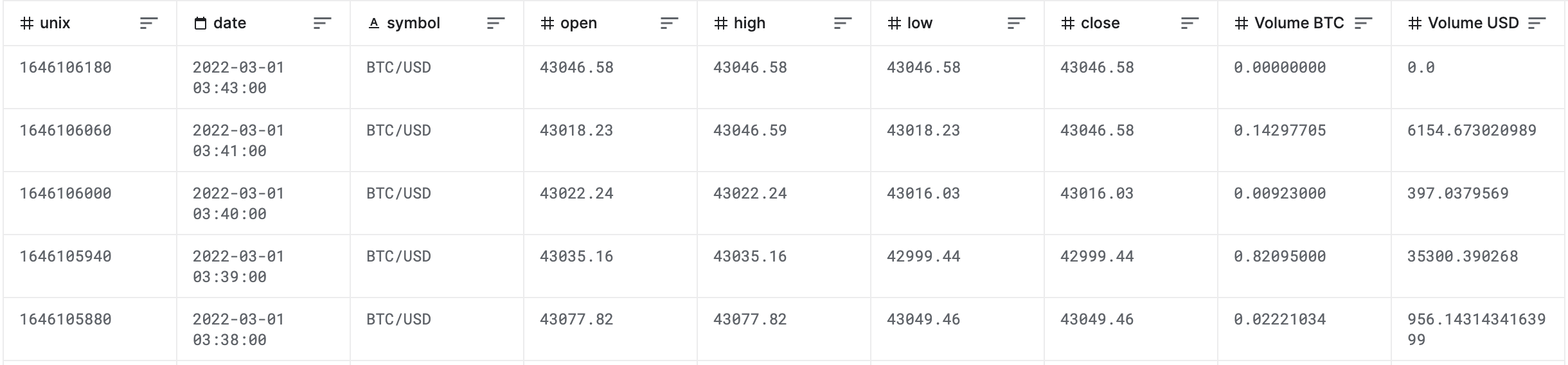``````CREATE TABLE `btc` (
`unix` bigint(20) NOT NULL,
`date` datetime NULL,
`symbol` varchar(30) NULL,
`open` decimalv3(8, 2) NULL,
`high` decimalv3(8, 2) NULL,
`low` decimalv3(8, 2) NULL,
`close` decimalv3(7, 2) NULL,
`Volume_BTC` decimalv3(10, 8) NULL,
`Volume_USD` decimalv3(38, 30) NULL
) ENGINE=OLAP
DUPLICATE KEY(`unix`)
COMMENT 'OLAP'
DISTRIBUTED BY HASH(`unix`) BUCKETS 4
PROPERTIES (
"replication_allocation" = "tag.location.default: 1"
);
``````

``````mysql> select avg(Volume_BTC),avg(Volume_USD),sum(Volume_BTC),sum(Volume_USD) from btc where to_date(date)='2022-01-01';
+-------------------+--------------------------------------+-------------------+-----------------------------------------+
| avg(`Volume_BTC`) | avg(`Volume_USD`)                    | sum(`Volume_BTC`) | sum(`Volume_USD`)                       |
+-------------------+--------------------------------------+-------------------+-----------------------------------------+
|        0.51494486 | 24236.665942788256243957638888888888 |      741.52060313 | 34900798.957615088991299000000000000000 |
+-------------------+--------------------------------------+-------------------+-----------------------------------------+
``````

### 效能對比

tpch1庫為DecimalV3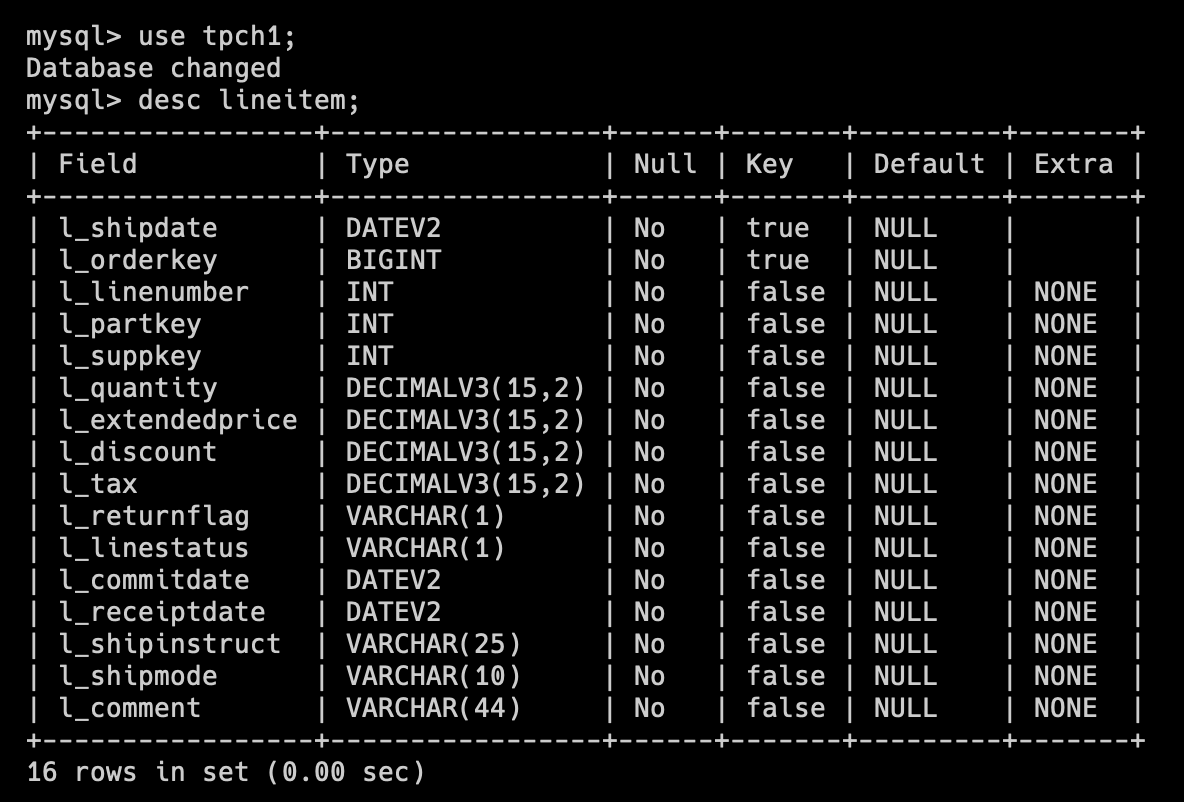tpch2庫為老版本Decimal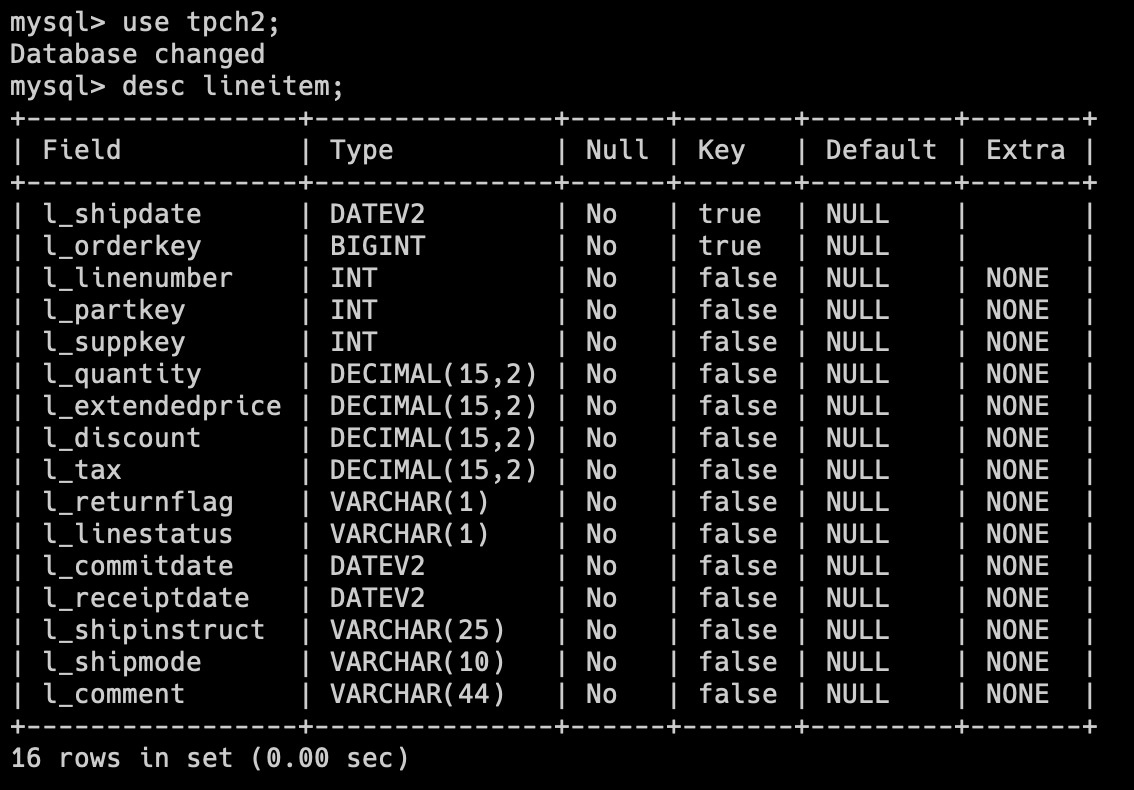#### 執行速度

SQL Q1

``````select /*+SET_VAR(exec_mem_limit=8589934592, parallel_fragment_exec_instance_num=16, enable_vectorized_engine=true, batch_size=4096, disable_join_reorder=false, enable_cost_based_join_reorder=false, enable_projection=false) */
l_returnflag,
l_linestatus,
sum(l_quantity) as sum_qty,
sum(l_extendedprice) as sum_base_price,
sum(l_extendedprice * (1 - l_discount)) as sum_disc_price,
sum(l_extendedprice * (1 - l_discount) * (1 + l_tax)) as sum_charge,
avg(l_quantity) as avg_qty,
avg(l_extendedprice) as avg_price,
avg(l_discount) as avg_disc,
count(*) as count_order
from
lineitem
where
l_shipdate <= date '1998-12-01' - interval '90' day
group by
l_returnflag,
l_linestatus
order by
l_returnflag,
l_linestatus;
``````

tpch1庫（DecimalV3）的 SQL 執行結果為 6.38s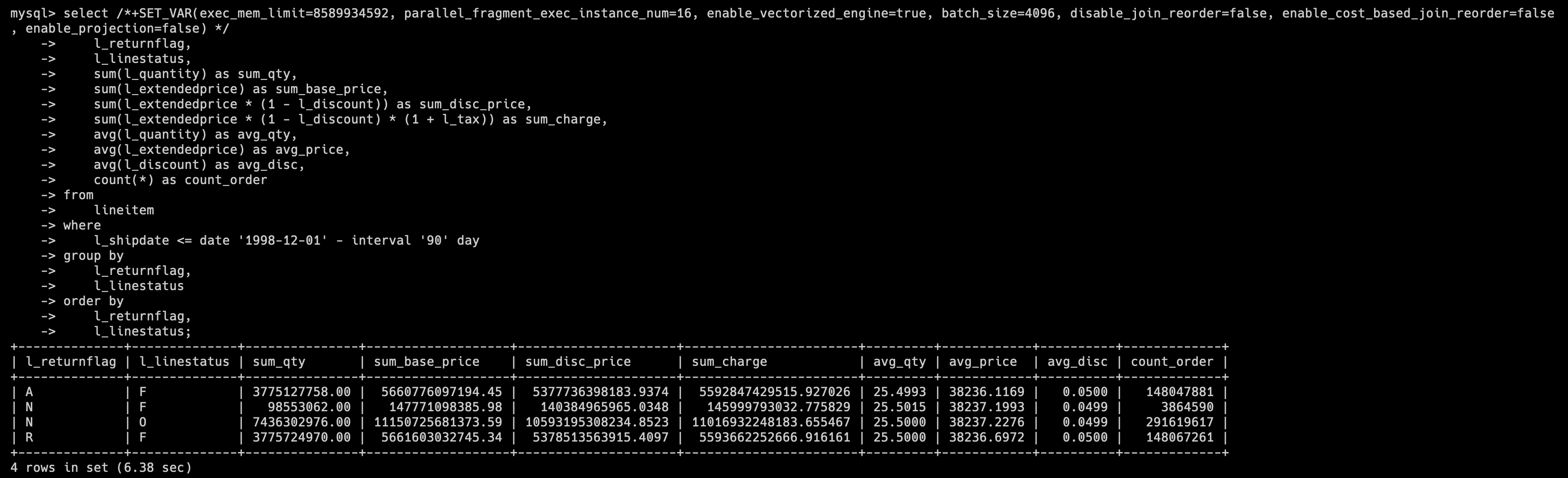tpch2 庫（老版本 Decimal）的 SQL 執行結果為 8.13s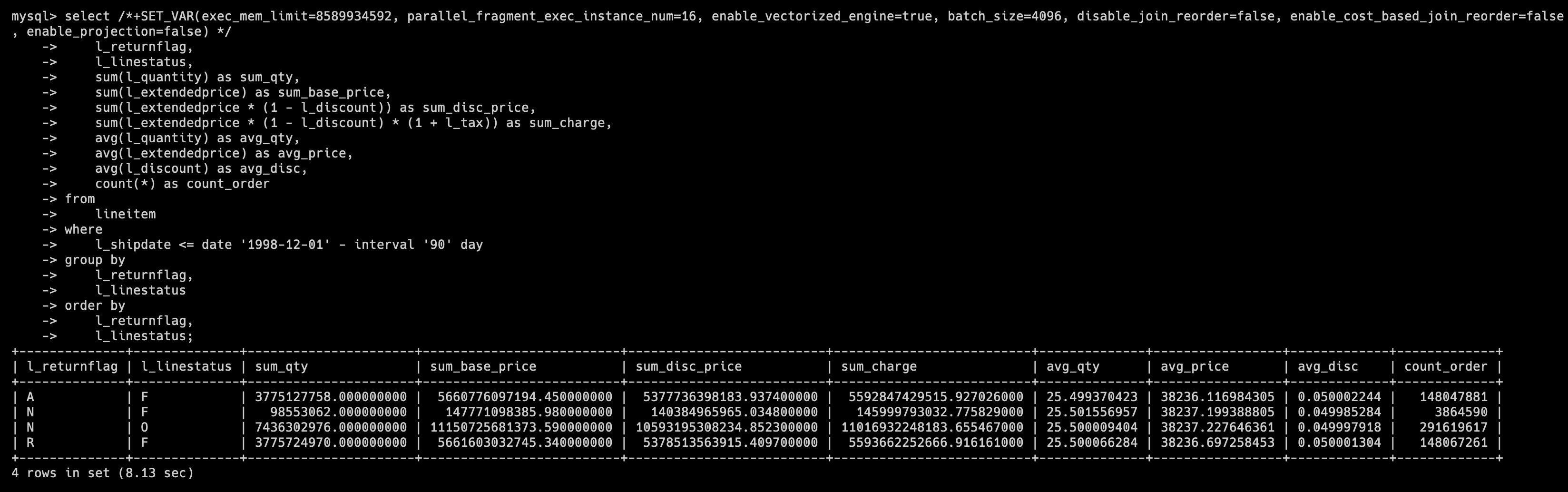SQL Q1 所查詢的表是上述展示欄位的表 Lineitem，我們可以看到在 DecimalV3 的情況下，查詢 速度較老版本有 27.4% 的提升。

#### 儲存佔用

tpch1庫（DecimalV3）的 Lineitem 表的儲存佔用為 18.475GB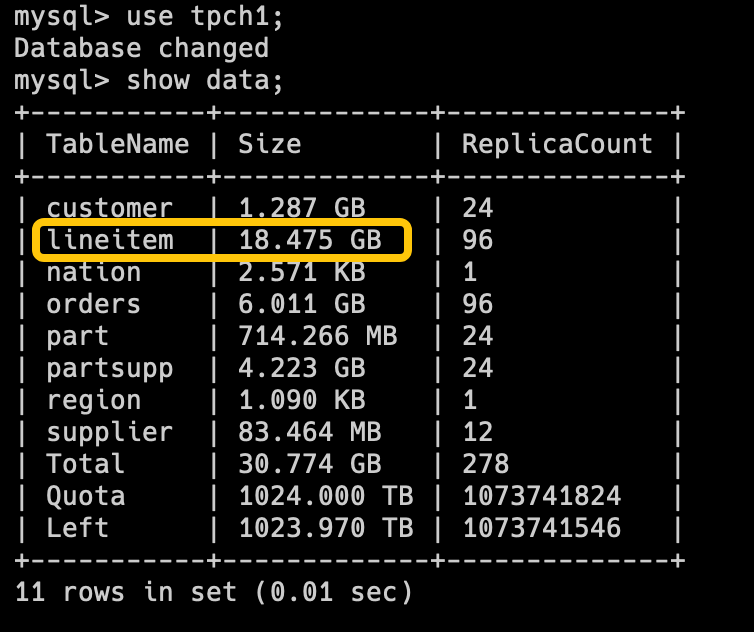tpch2 庫（老版本 Decimal）的 Lineitem 表的儲存佔用為 20.893GB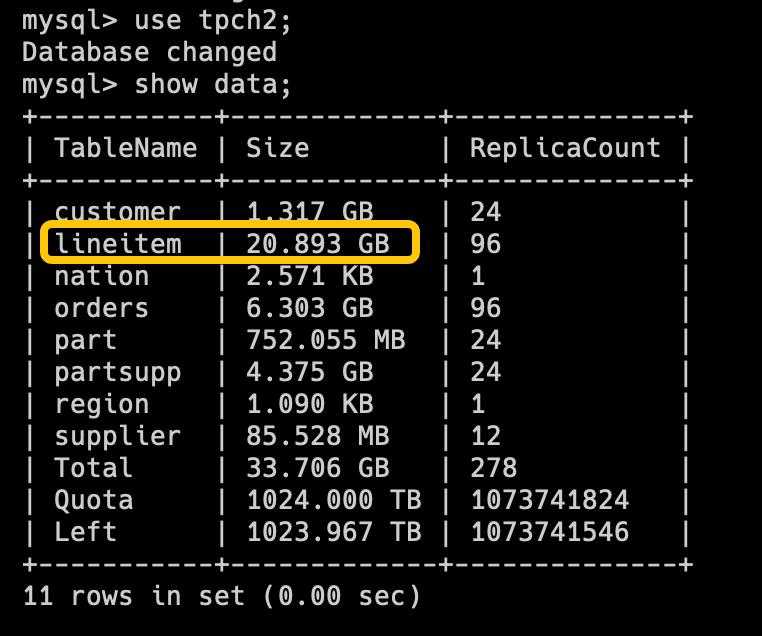#### 記憶體佔用

``````select count(*)
from
(   select l_quantity,l_extendedprice,l_discount,l_tax
from lineitem
where l_shipdate < '1995-01-01'
group by l_quantity,l_extendedprice,l_discount,l_tax
)tmp;
``````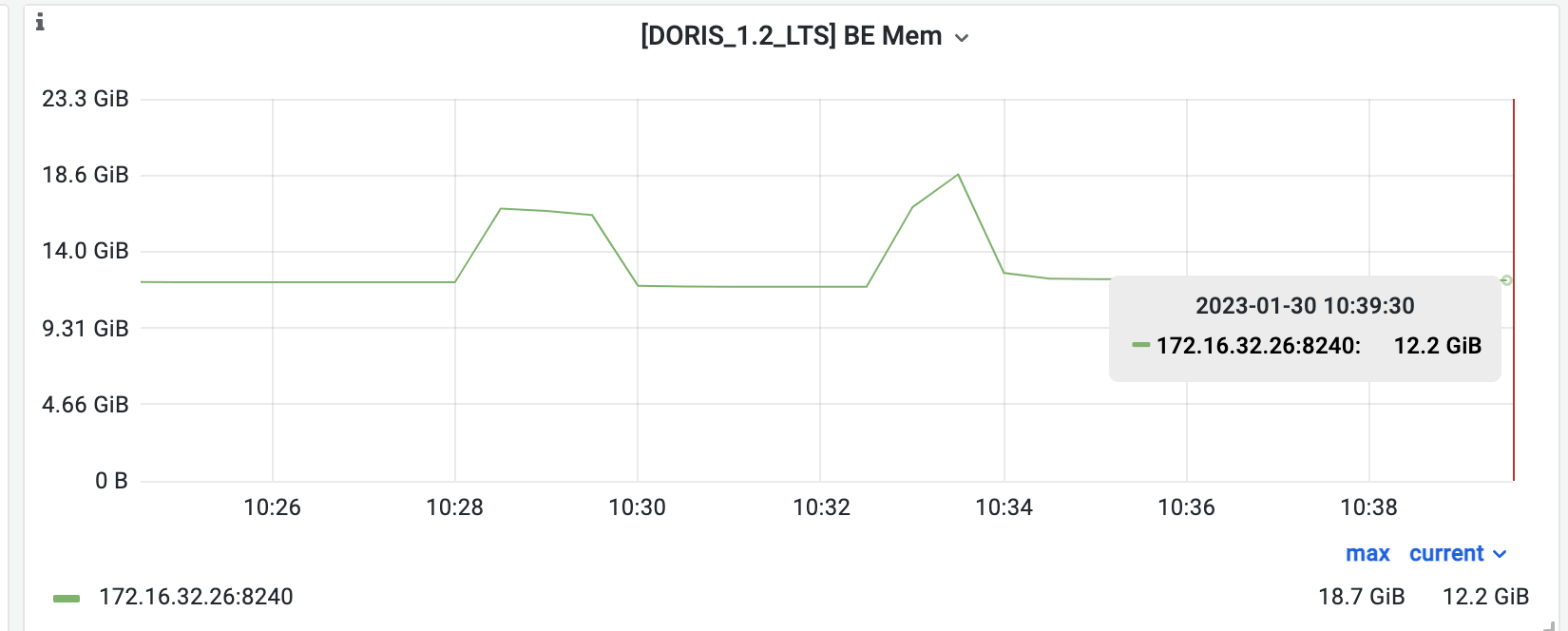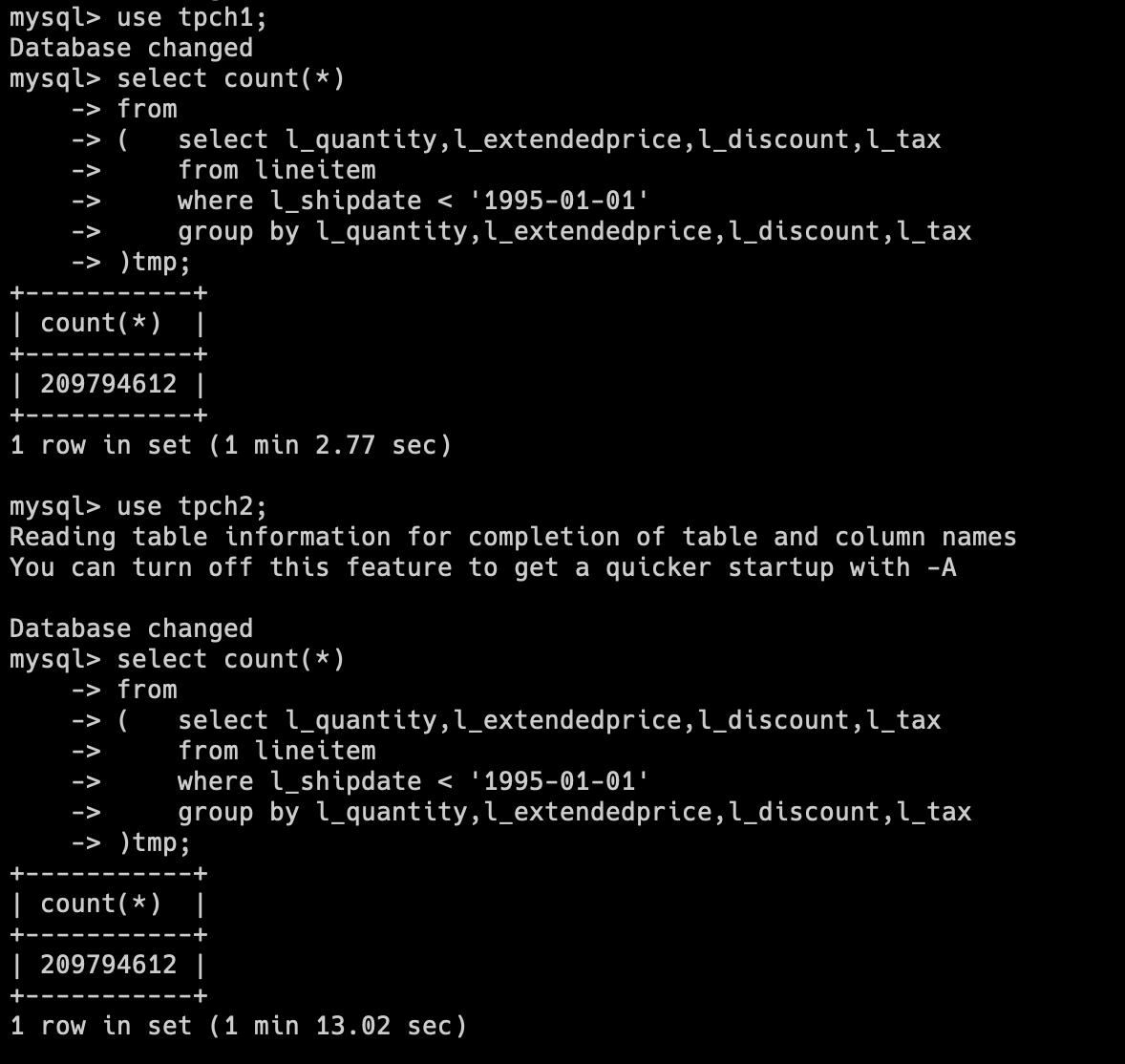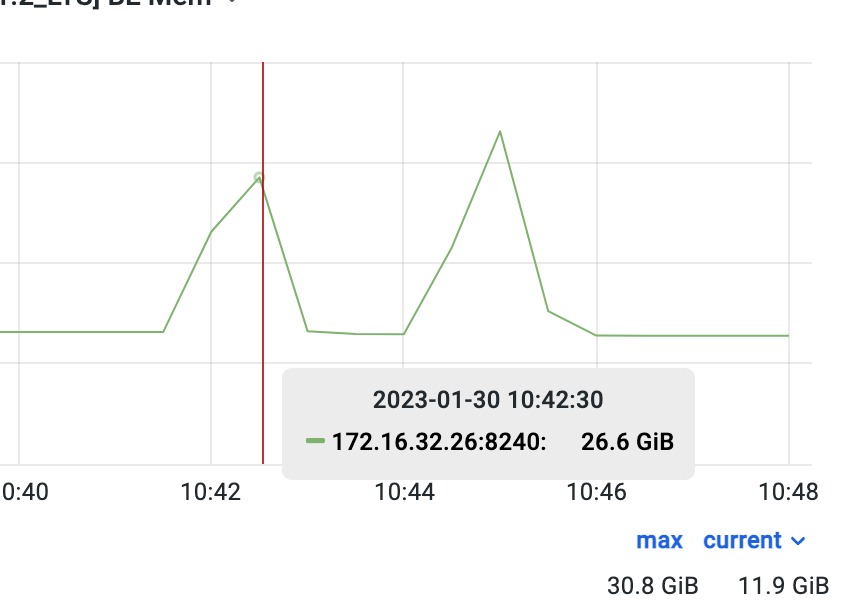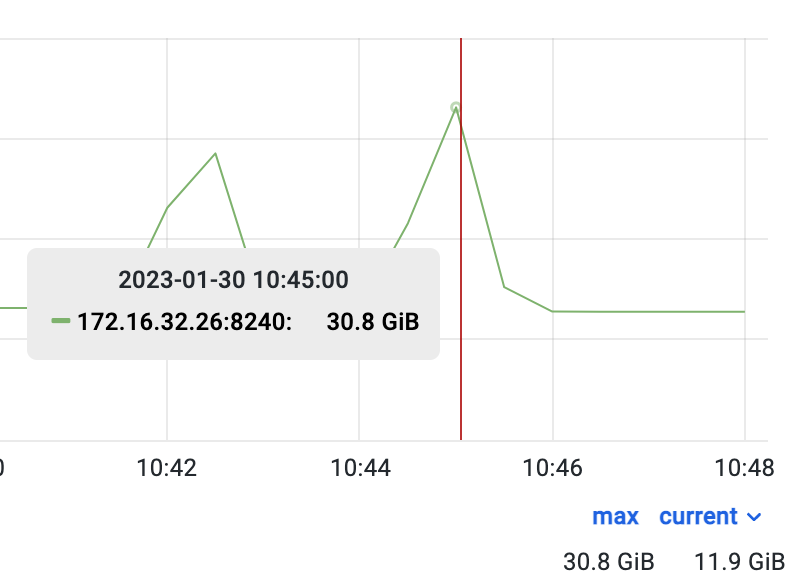### 總結

Apache Doris 1.2.1 版本推出的 DecimalV3 實現了更高的精度，更高的效能，更完備的精度推演，使得 Doris 更加適用於金融財務、科學計算等有精確計算需求的應用場景，結合 Apache Doris 強大的分析計算效能，給相關使用者及行業提供了更準確、完善的資料服務。